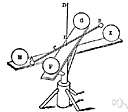# gravitational constant

Also found in: Thesaurus, Medical, Financial, Encyclopedia, Wikipedia.

## gravitational constant

n. Abbr. G
The constant relating the force of gravitational attraction between two bodies to the product of their masses and the inverse square of the distance between them in Newton's law of gravitation. It equals 6.67 × 10-11 m3kg-1s-2.
American Heritage® Dictionary of the English Language, Fifth Edition. Copyright © 2016 by Houghton Mifflin Harcourt Publishing Company. Published by Houghton Mifflin Harcourt Publishing Company. All rights reserved.

## gravitational constant

n
(General Physics) the factor relating force to mass and distance in Newton's law of gravitation. It is a universal constant with the value 6.673 × 10–11 N m2 kg–2. Symbol: G
Collins English Dictionary – Complete and Unabridged, 12th Edition 2014 © HarperCollins Publishers 1991, 1994, 1998, 2000, 2003, 2006, 2007, 2009, 2011, 2014

## grav·i·ta·tion·al constant

(grăv′ĭ-tā′shə-nəl)
A number used to calculate the force of the gravitational attraction between two bodies in Newton's law of gravitation. The gravitational constant equals 6.67 × 10-11 cubic meters per kilogram per second squared. See more at Newton's law of gravitation.
The American Heritage® Student Science Dictionary, Second Edition. Copyright © 2014 by Houghton Mifflin Harcourt Publishing Company. Published by Houghton Mifflin Harcourt Publishing Company. All rights reserved.
ThesaurusAntonymsRelated WordsSynonymsLegend:
 Noun 1gravitational constant - (physics) the universal constant relating force to mass and distance in Newton's law of gravitationlaw of gravitation, Newton's law of gravitation - (physics) the law that states any two bodies attract each other with a force that is directly proportional to the product of their masses and inversely proportional to the square of the distance between themnatural philosophy, physics - the science of matter and energy and their interactions; "his favorite subject was physics"constant - a number representing a quantity assumed to have a fixed value in a specified mathematical context; "the velocity of light is a constant"
Based on WordNet 3.0, Farlex clipart collection. © 2003-2012 Princeton University, Farlex Inc.
Translations
Gravitationskonstante
References in periodicals archive ?
As this behavior was similar to devices (a rotor and torsion balance or a device for measuring tiny forces and torques) used for measuring gravitational constant and density of Earth, the researchers posited that the wide-spanning uses of the newly-developed object could also include a study of quantum mechanics as well as the properties of a vacuum 6 both of which are not well understood.
Precision measurement of the Newtonian gravitational constant using cold atoms.
Take for example the simple Law of Gravity envisioned by Newton in 1660s that force of gravity equals the product of masses divided by the square of the distance between the two masses and multiplied by a gravitational constant. This very simple but prophetic mathematical formulation has been providing the basis for precisely calculating the trajectories of all space missions, even beyond the solar system, undertaken to-date.
[M.sub.gj] is gravitational mass of mass j, G(t) is the gravitational constant in time t and [R.sub.ij] is the distance between the two objects j and i.
We assume Planck constant, Boltzmann constant, and the speed of light as h = [K.sub.B] = c = 1 and define the gravitational constant 8[pi]G by the relation [K.sup.2] = 8[pi]/[M.sup.2.sub.pl] (where [M.sub.pl] is Planckmass).
Drawn from the proceedings of the XXII International Conference on Laser Spectroscopy (ICOL2015), held in Singapore in June and July of 2015, the eight papers in this volume discuss quantum walks with neutral atoms in state-dependent optical lattices; the role of muonic atoms in nuclear structure; the development and optimization of Doppler broadening thermometry using acetylene as a molecular target; antiferromagnetism with ultracold atoms; the generation and transfer of stable optical frequencies in optical clocks with trapped ions; precision measurement of the Newtonian gravitational constant by atom interferometry; optical sideband cooling in a Penning trap; and Bose-Einstein condensation of photons vs.
It can speed up the solution process by adjusting the accuracy of the search with gravitational constant. Furthermore, GSA is memoryless and works efficiently similar to algorithms with memory.
where [M.sub.i](t) and [M.sub.j](t) are the masses of the ith agent and the jth agent, respectively, G(t) is the gravitational constant at the ith time, [epsilon] is a small constant, and [R.sub.ij] is the Euclidian distance between the ith agent and the jth agent .
where: G is the gravitational constant, M and m are the masses of the two objects, r is the distance between the two objects, c is the speed of light, p is the half normal chord for the object m moving around the object M along with a curve, and the value of p is given by: p = a(l-[e.sup.2]) (for ellipse), p = a ([e.sup.2]-l) (for hyperbola), p = [y.sup.2]/2x (for parabola).
where [rand.sub.j] is a random number in the interval [0, 1], G(t) is the gravitational constant at time t, [M.sub.i] and [M.sub.j] are masses of agents i and j, [epsilon] is a small value and [R.sub.ij] (t) is the Euclidean distance between two agents, i and j.

Site: Follow: Share:
Open / Close